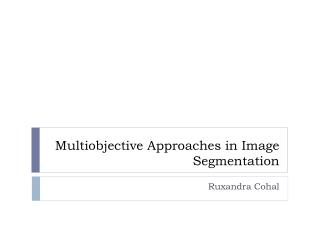DownloadDownload PresentationMultiobjective Approaches in Image Segmentation

# Multiobjective Approaches in Image Segmentation

Download Presentation## Multiobjective Approaches in Image Segmentation

- - - - - - - - - - - - - - - - - - - - - - - - - - - E N D - - - - - - - - - - - - - - - - - - - - - - - - - - -
##### Presentation Transcript

1. Multiobjective Approaches in Image Segmentation RuxandraCohal

2. Keywords Image segmentation Multiobjective Optimization Image analysis Thresholding Pixel classification

3. Image segmentation techniques results http://www.cs.toronto.edu/~jepson/csc2503/segmentation.pdf

4. Image segmentation • image partitioning • similarity/dissimilarity criteria • challenging task of image processing • influence over the result of image analysis

5. Multipleobjective Optimization • single objective optimization problem • goal: minimize, maximize, achieve a certain value • multiobjective optimization problem • goal: optimize in the same time a certain number of criteria

6. Multipleobjective Optimization • multiobjective optimization criteria examples: • minimize overall deviation • maximize connectivity • minimize the number of features • minimize the error rate of the classifier

7. Multiobjective Optimization Methods • Reduce the MO function to a single-objective function • assign a numerical weight to each objective • combine the values of the weighted criteria into a single value

8. Multiobjective Optimization Methods 2. Simultaneous optimization of several objectives • Pareto approach • dominance relation • Pareto-optimal set = a set of solutions that are non-dominated with respect to each other • Pareto optimal solution sets are preferred to single solutions

9. Image segmentation techniques • Image thresholding • region segmentation method • popular approach due to its straightforward implementation • challenge: automatic fitting of threshold

10. Image segmentation techniques • Image thresholding • MO approach: combination of segmentation objectives of two thresholding techniques: • Otsu’s method • Gaussian curve fitting method • the objective functions are optimized using: • weighted sum of within-class criterion • overall probability of error criterion

11. Image segmentation techniques • Pixel classification - supervised classification • training set of data • classifier • number of classes • numerical characteristics (mean, variance etc.)

12. Image segmentation techniques • Pixel classification - supervised classification • MO optimization methods can be used to design classifiers • the objective functions are usually related to: • rules definition of the classifiers • error rate of the classifier • diversity measurement

13. Image segmentation techniques • Pixel classification - unsupervised classification • clustering in image segmentation = the process of identifying groups of similar image primitives • image primitives: • pixels • regions • line elements

14. Image segmentation techniques • Pixel classification - unsupervised classification • the lack of a precise definition for what a cluster is result in a large number of clustering algorithms • clustering algorithms can find structures (partitions) at different levels of refinement

15. Image segmentation techniques • Pixel classification - unsupervised classification • from MO perspective, the optimization/search techniques used are divided into • deterministic: exhaustive enumeration • stochastic: generate a near-optimal partition reasonably quickly

16. Image segmentation techniques • Pixel classification - unsupervised classification • stochastic search technique: evolutionary computation approaches • chromosomes(candidate solutions): coordinates of centers of the partitions • evolutionary operators: selection, recombination, mutation • fitness function: objective function • multiobjective clustering algorithms: • NSGA (2 objectives), NSGA-II(3 objectives) • PESA(2 objectives)

17. Image segmentation techniques • Pixel classification - unsupervised classification • other approaches • PSO (Particle Swarm Optimization) • SA (Simulated Annealing) • Hill Climbing and Genetic Algorithms

18. Conclusions • Image segmentation • complex process • involves a set of objective to be optimized • the multipleobjective optimization approach facilitate the use of many types of algorithms

19. Bibliography • Multiobjective Optimization Approaches in Image Segmentation – The Directions and Challenges – Bong Chin-Wei, MandavaRajeswari